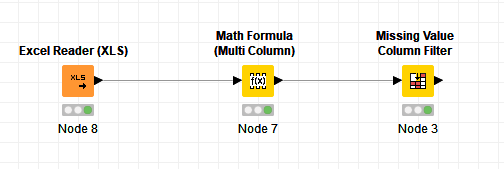# Filtering many columns based on values

Hello,

I have a large dataset (10.000+ columns and 4000 rows), filled with Strings represented as 0’s and 1’s only.
Now I’d like to remove every column that contains less than a certain amount of 1’s (less than 10% or less than 400 occurences for example).

Is there a node that does what I’m looking for?

Thanks,
Michael

hello Michael

to me it looks a bit odd to have more colums than rows… so if possible and reasonable a pivot might be helpful…
then I would recommend to have the 0 and 1 imported as numbers not as string… or to convert them to numbers with the node “string to number”… maybe in a loop if it is so huge…
once converted you can do a group by and calculate the sum…
knowing the total rows (count) and having the sum, gives you the relation of how many 1 are in the column…
and therfore you can filter out the columns that have less than 10% of total or less than 400 as you like…

1 Like

I hoped there was a single node that I simply didn’t find but your answer was really helpful.

Hi @mtest -

If you are able to read your values in as numbers instead of strings, there’s a way to do this using only two nodes. I used a Math Formula (Multi Column) node to divide by the number in each cell. Since dividing by zero produces a missing value, I then used a Missing Value Column Filter node to remove columns with percentage of missing values above a given threshold.

This is very hacky and there’s almost certainly a better way to do it! But maybe it will help you.

MissingValueColumnFilterExample.knwf (8.5 KB)
Testsheet.xlsx (8.7 KB)3 Likes

It could be slow but try.

1. Transpose table.
2. Use column aggregator with concatenate.
3. String Manipulation with count(concat String, “1”)
4. Filter rows with say 400 or higher
5. Delete column with counts if necessary
6. Transpose table
1 Like

This topic was automatically closed 7 days after the last reply. New replies are no longer allowed.